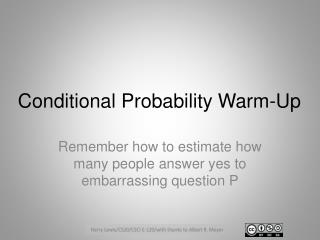Download PresentationConditional Probability Warm-Up

# Conditional Probability Warm-Up - PowerPoint PPT PresentationDownload Presentation## Conditional Probability Warm-Up

- - - - - - - - - - - - - - - - - - - - - - - - - - - E N D - - - - - - - - - - - - - - - - - - - - - - - - - - -
##### Presentation Transcript

1. Conditional Probability Warm-Up Remember how to estimate how many people answer yes to embarrassing question P

2. Calculating Pr(P) from Pr(H∪P) H P Because H and P are independent events, Pr(H∩P) = Pr(H) ∙ Pr(P). Pr(H) = .5 Pr(H∪P) = Pr(H) + Pr(P) - Pr(H∩P). So Pr(P) = Pr(H∪P) - Pr(H) + Pr(H)∙Pr(P) Pr(P) = Pr(H∪P) -.5 + .5 ∙ Pr(P) Pr(P) = 2 ∙ Pr(H∪P) – 1

3. Now Suppose You Raise Your Hand:How Suspicious Should I Be of You? That is, what is Pr(P | H∪P)? Let R = H∪P, r = Pr(R), p = Pr(P) = 2r-1 We want Pr(P | R) = Pr(P∩R)/Pr(R) But P∩R = P∩(H∪P) = P So Pr(P∩R)/Pr(R) = Pr(P)/Pr(R) = p/r = (2r-1)/r = 2 – 1/r If r = ¾, Pr(P|R) = 2 – 4/3 = 2/3

4. Important Lessons! So if r = .5, Pr(P|R) = 0; if r = 1, Pr(P|R) = 1 With only a finite sample, impossible to calculate probabilitiesprecisely

5. FINIS